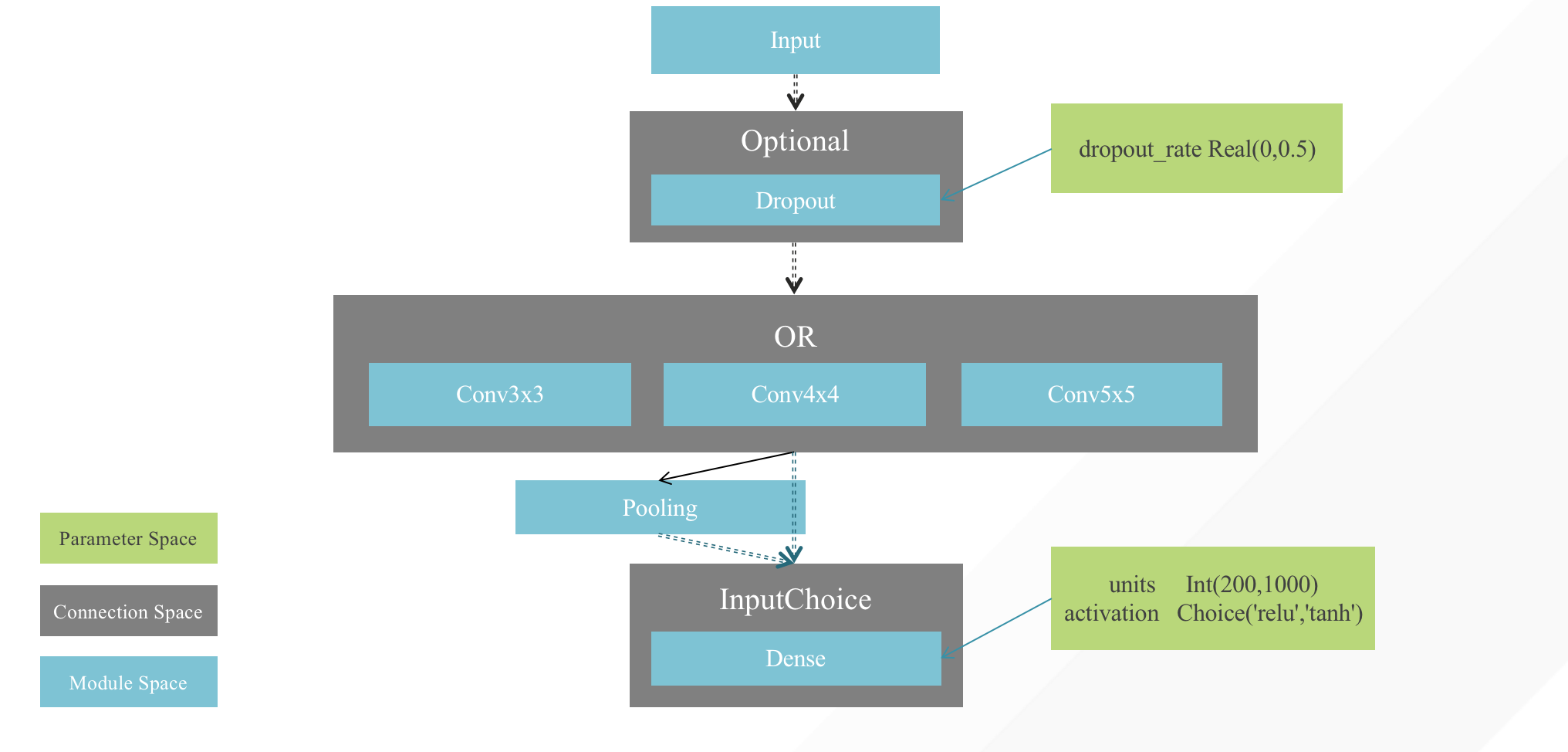# Search Space¶

For hyper-parameter optimization, the search space might be a closed subset of Euclidean space composed of discrete or continuous values. For neural architecture search(NAS), The network architecture is composed of graphs generated by various layers through connections, and all feasible graphs constitute the search space.

Hypernets introduced an abstract search space representation and taken into account both of the above requirements, so that it can use a unified primitive to define various search spaces.

• illustration of the search space in Hypernets## HyperSpace¶

The general form of `HyperSpace` is a graph formed by connecting nodes and edges, each node can contain a set of hyper-parameters. `HyperModel` can represent a pipeline, or a more complex directed acyclic graph(DAG), in some cases The objective function to be optimized has only one node, for example when we only need to optimize a few hyper-parameters of a lightgbm model.

## Parameter Space¶

ParameterSpace is diveded into two types: mutable and immutable. The mutable is created with a set of possible values, for example, `Real(0.1, 0.9)`, `Choice(['relu','tanh'])`, etc. The immutable includes `Constant`,`Dynamic`, and `Cascade`.

• Mutable: `Int`, `Real`, `Bool`, `Choice`, `MultipleChoice`
• Immutable: `Constant`, `Dynamic`, `Cascade`

## Connection Space¶

ConnectionSpace is used to represent possible connections between modules.

• Optional: Whether to insert a module between two modules.```from hypernets.frameworks.keras.layers import Dense, Input, Dropout
from hypernets.core.ops import Optional

module_a = Input()
module_b = Dropout()
optional_dropout = Optional(module_b, keep_link=True)(module_a)
module_c = Dense()(optional_dropout)
```
• ModuleChoice: There are several optional modules on a node, choose one of them.```from hypernets.frameworks.keras.layers import Input, BatchNormalization
from hypernets.core.ops import ModuleChoice

module_a = Input()
or_conv_pool = ModuleChoice([sepconv5x5(),sepconv3x3(),avgpooling3x3()])(module_a)
module_c = BatchNormalization()(or_conv_pool)
```
• Sequential: A series of modules connected in order.```from hypernets.frameworks.keras.layers import Dense, Input, BatchNormalization
from hypernets.core.ops import Sequential

module_a = Input()
module_b = Dense()
module_c = BatchNormalization()
Sequential([module_a,module_b,module_c])
```
• Permutation: Choose one of permutations of a module list and connected in order.```from hypernets.frameworks.keras.layers import Dense, BatchNormalization, Dropout, Activation
from hypernets.core.search_space import Choice
from hypernets.core.ops import Permutation, Sequential, Optional

dense = Dense(units=Choice([100, 300, 500, 1000]))
act = Activation(activation=Choice(['relu', 'tanh']))
optional_bn = Optional(BatchNormalization(), keep_link=True)
dropout = Dropout(rate=Choice([0, 0.1, 0.2, 0.3, 0.5]))

# Use `Permutation` to try different arrangements of act, optional_bn, dropout
# optional_bn is optional module and will be skipped when hp_use_bn is False
perm_act_bn_dropout = Permutation([act, optional_bn, dropout])

# Use `Sequential` to connect dense and perm_act_bn_dropout in order
seq = Sequential([dense, perm_act_bn_dropout])
```
• Repeat: The module is repeated n times and connected in order.```from hypernets.frameworks.keras.layers import Dense, BatchNormalization, Dropout, Activation
from hypernets.core.search_space import Choice
from hypernets.core.ops import Permutation, Sequential, Optional, Repeat

dense = Dense(units=Choice([100, 300, 500, 1000]))
act = Activation(activation=Choice(['relu', 'tanh']))
optional_bn = Optional(BatchNormalization(), keep_link=True)
dropout = Dropout(rate=Choice([0, 0.1, 0.2, 0.3, 0.5]))

# Use `Permutation` to try different arrangements of act, optional_bn, dropout
# optional_bn is optional module and will be skipped when hp_use_bn is False
perm_act_bn_dropout = Permutation([act, optional_bn, dropout])

repeat_seq = Repeat(module_fn=lambda :Sequential([dense, perm_act_bn_dropout]), repeat_times=Choice([2,3,4]))
```
• InputChoice: A modules has n upstream modules, choose one or more connections.```from hypernets.frameworks.keras.layers import Input,Dense, BatchNormalization, Dropout, Activation
from hypernets.core.search_space import Choice
from hypernets.core.ops import Permutation, Sequential, Optional, Repeat, InputChoice

module_a = Input()
module_b = Input()
module_c = Input()
ic = InputChoice(inputs=[module_a,module_b,module_a], num_chosen_most=2)([module_a,module_b,module_c])
add = Add()(ic)
```

## Module Space¶

ModuleSpace represents an optimizable function with a set of hyper-parameters. Unlike ParameterSpace and ConnectionSpace, ModuleSpace is related to frameworks or libraries. In different `HyperModel`, it is necessary to define a corresponding series of ModuleSpace.